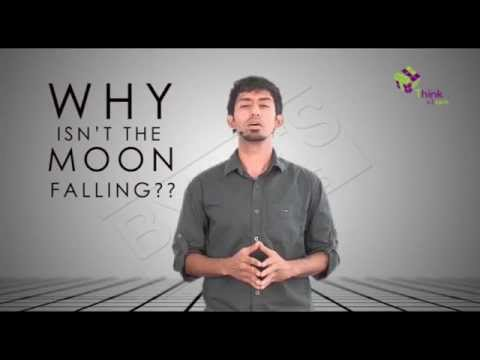# Acceleration Due To Gravity

## Introduction to GravityEarth’s acceleration due to gravity acts on every object on earth and produces a force on it that acts towards the centre of the earth.

Introduction to Gravity:

• It is a common belief that an apple fell on Newton’s head and he realized that gravity exists. This isn’t completely incorrect. But Newton already knew about the existence of gravity. He was just trying to find out if the gravitational pull of the earth was what was holding the moon in its orbit.
• Since the apple fell perpendicular to the earth (and not in any other direction) and gathered up speed as it fell, he realized that it obviously was being accelerated at some rate. This rate of acceleration was acceleration due to gravity.

### Acceleration:

Acceleration is caused by force when a net force acts on a body and keeps acting in the same direction, the body will accelerate in that direction. One force that always acts on the bodies that have mass is gravity, if we consider the force of gravity acting on a body excluding the air resistance, the body should theoretically accelerate towards earth at the rate of 9.81 m/ $s^2$.

### Force acting on a body due to gravity is given by:

f = mg

Where f is the force acting on the body,

g is the acceleration due to gravity,

m is mass of the body.

According to the universal law of gravitation,

f = $\frac {GmM}{(r+h)^2}$

Where, f is the force between two bodies,

G is the universal gravitational constant ( $6.67\times 10^{-11} N.m^2/kg^2$ )

m is the mass of the object,

M is the mass of the earth,

r is the radius of the earth.

h is the height at which the body is from the surface of the earth,

As h is negligibly small compared to the radius of earth we reframe the equation as follows

f = $\frac {GmM}{r^2}$

Now equating both the expressions,

mg = $\frac {GmM}{r^2}$

g = $\frac {GM}{r^2}$

This is the expression for acceleration due to gravity; it depends on the Mass of earth and radius of the earth. This helps us understand the following:

1. All bodies experience the same acceleration due to gravity irrespective of its mass.
2. The gravity value on earth depends upon the mass of the earth and not the mass of the object. (because the mass of the object is negligibly small compared to the mass of earth)

Value of gravity g on earth is about 9.81 m/ $s^2$ .

Since acceleration due to gravity is a vector quantity, it has to have both direction and magnitude. The direction is always towards the body in consideration and the magnitude varies on different celestial bodies. For example, the acceleration due to gravity on the moon is $1.62 ~ m/s^2$ and on Jupiter it is around $26 ~m/s^2$ .

An experiment was conducted in NASA’s Space Simulation chamber in Ohio where a bowling ball and a feather were dropped together in a vacuum. Which object do you think reached the ground first? Since there is no other force (usually in such a case, the feather would experience more air resistance than the ball), both the objects reached the ground at the same time.

You may have heard of a variation of this dialogue said somewhere “The mass of your body is the same wherever you go but your weight may vary”. For example, if you are around 50 kg on earth you will be 50 kg on the moon as well. But on earth, your weight will be 490 N and on the moon it will be 381 N. Now, how did we arrive on that?

Hint: Try usingNewton’s second law of motion!

There’s no better way to learn concepts than by applying them. Stay tuned with Byju’s to learn more about gravity, laws of motion and much more.#### Practise This Question

Which one of the following graphs represents correctly the variation of the gravitational field (I) with the distance (r) from the centre of a spherical shell of mass M and radius a